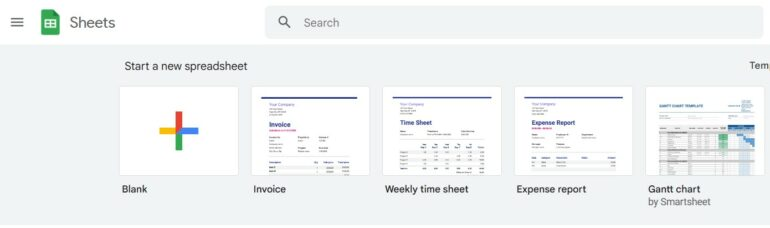Number 1. Understanding Formulas and Functions

Formulas and functions are the building blocks of Google Sheets. A formula is a calculation that can be performed based on the data in your spreadsheet. A function, on the other hand, is a pre-written formula that performs a specific task. To use a function, you simply need to enter its name, followed by the arguments that it requires, into a cell.

Number 2. Common Formulas

Google Sheets provides a wide range of formulas that you can use to perform various calculations on your data. Some of the most commonly used formulas include SUM, AVERAGE, MIN, and MAX. These formulas can be used to calculate the sum, average, minimum, and maximum values of a range of cells, respectively.

For example, to calculate the sum of the values in cells A1 to A5, you would enter the following formula into a cell: =SUM(A1:A5). Similarly, to calculate the average of the values in cells A1 to A5, you would enter the following formula into a cell: =AVERAGE(A1:A5).

In addition to the common formulas, Google Sheets also provides a wide range of advanced formulas that you can use to perform more complex calculations. Some of the most commonly used advanced formulas include IF, VLOOKUP, HLOOKUP, INDEX, and MATCH.

The IF formula is a conditional formula that allows you to perform calculations based on certain conditions. For example, if you wanted to calculate a grade for a test score, you could use the following formula: =IF(A1>=90, “A”, “B”).

The VLOOKUP and HLOOKUP formulas are used to look up data from a table based on a specific value. For example, if you have a table of grades and corresponding grades, you could use the following formula to look up the grade for a test score: =VLOOKUP(A1,A2:B6,2,FALSE).

The INDEX and MATCH formulas can be used together to look up data from a table based on both a row and column value. For example, if you have a table of grades and corresponding grades, you could use the following formula to look up the grade for a test score: =INDEX(B2:B6,MATCH(A1,A2:A6,0)).

Number 4. Functions

Google Sheets provides a wide range of functions that you can use to perform various calculations and data analysis tasks. Some of the most commonly used functions include SUMIF, COUNTIF, and AVERAGEIF.

The SUMIF function is used to sum data based on specific conditions. For example, if you wanted to sum the values in cells A1 to A5 that are greater than 10, you could use the following formula: =SUMIF(A1:A5, “>10”).

The COUNTIF function allows you to count the number of cells in a range that meet a specified criteria. To use the COUNTIF function, simply enter “=COUNTIF(range, criteria)” into a cell in your Google Sheet. Replace “range” with the cells that you want to count and “criteria” with the condition that must be met for a cell to be counted. For example, if you wanted to count the number of cells in the range A1:A10 that contain the value “apple”, you would enter “=COUNTIF(A1:A10, “apple”)” into a cell in your Google Sheet.

The AVERAGEIF function works similarly to the COUNTIF function, but instead of counting cells, it calculates the average of cells that meet a specified criteria. To use the AVERAGEIF function, enter “=AVERAGEIF(range, criteria)” into a cell in your Google Sheet. Replace “range” with the cells that you want to average and “criteria” with the condition that must be met for a cell to be included in the average calculation. For example, if you wanted to calculate the average of all cells in the range A1:A10 that contain the value “apple”, you would enter “=AVERAGEIF(A1:A10, “apple”)” into a cell in your Google Sheet.